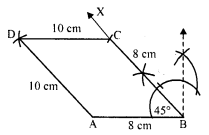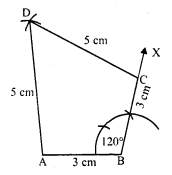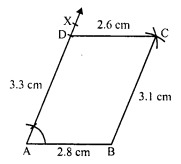## RD Sharma Class 8 Solutions Chapter 18 Practical Geometry Ex 18.3

These Solutions are part of RD Sharma Class 8 Solutions. Here we have given RD Sharma Class 8 Solutions Chapter 18 Practical Geometry Ex 18.3

Other Exercises

Question 1.
Construct a quadrilateral ABCD in which AB = 3.8 cm, BC = 3.4 cm, CD = 4.5 cm, AD = 5 cm and ∠B = 80°.
Solution:
Steps of construction :
(i) Draw a line segment AB = 3.8 cm.
(ii) At B, draw a ray BX making an angle of 80° and cut off BC = 3.4 cm.
(iii) With centre A and radius 5 cm and with centre C and radius 4.5 cm, draw arcs which intersect each other at D.Question 2.
Construct a quadrilateral ABCD given that AB = 8 cm, BC = 8 cm, CD = 10 cm, AD = 10 cm and ∠A = 45°.
Solution:
Steps of construction :
(i) Draw a line segment AB = 8 cm.(ii) At A, draw a ray AX making an angle of 45° and cut off BC = 8 cm.
(iii) With centre A and C and radius 10 cm, draw arcs intersecting each other at D.
Then ABCD is the required quadrilateral.

Question 3.
Construct a quadrilateral ABCD in which AB = 7.7 cm, BC = 6.8 cm, CD = 5.1 cm, AD = 3.6 cm and ∠C = 120°.
Solution:
Steps of construction :
(i) Draw a line segment BC = 6.8 cm.(ii) At C, draw a ray CX making an angle of 120° and cut off CD = 5.1 cm.
(iii) With centre B and radius 7.7 cm and with centre D and radius 3.6 cm draw arcs which intersect each other at A.
Then ABCD is the required quadrilateral.

Question 4.
Construct a quadrilateral ABCD in which AB = BC = 3 cm, AD = CD = 5 cm and ∠B = 120°
Solution:
Steps of construction :
(i) Draw a line segment AB = 3 cm.(ii) At B, draw a ray BX making an angle of 120° and cut off BC = 3 cm.
(iii) With centres A and C, and radius 5 cm, draw arcs intersecting each other at D.
Then ABCD is the required quadrilateral.

Question 5.
Construct a quadrilateral ABCD in which AB = 2.8 cm, BC = 3.1 cm, CD = 2.6 cm and DA = 3.3 cm and ∠A = 60°.
Solution:
Steps of construction :
(i) Draw a line segment AB = 2.8 cm.(ii) At A draw a ray AX making an angle of 60° and cut off AD = 3.3 cm.
(iii) With centre B and radius 3.1 cm and with centre D and radius 2.6 cm, draw arc which intersect each other at C.
(iv) Join CB and CD.
Then ABCD is the required quadrilateral.

Question 6.
Construct a quadrilateral ABCD in which AB = BC = 6 cm, AD = DC = 4.5 cm and ∠B = 120°.
Solution:
Steps of construction:
The construction is not possible to draw as arcs of radius 4.5 cm from A and C, do not intersect at any point.Hope given RD Sharma Class 8 Solutions Chapter 18 Practical Geometry Ex 18.3 are helpful to complete your math homework.

If you have any doubts, please comment below. Learn Insta try to provide online math tutoring for you.